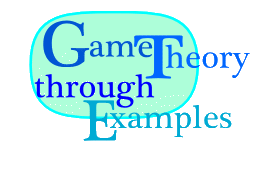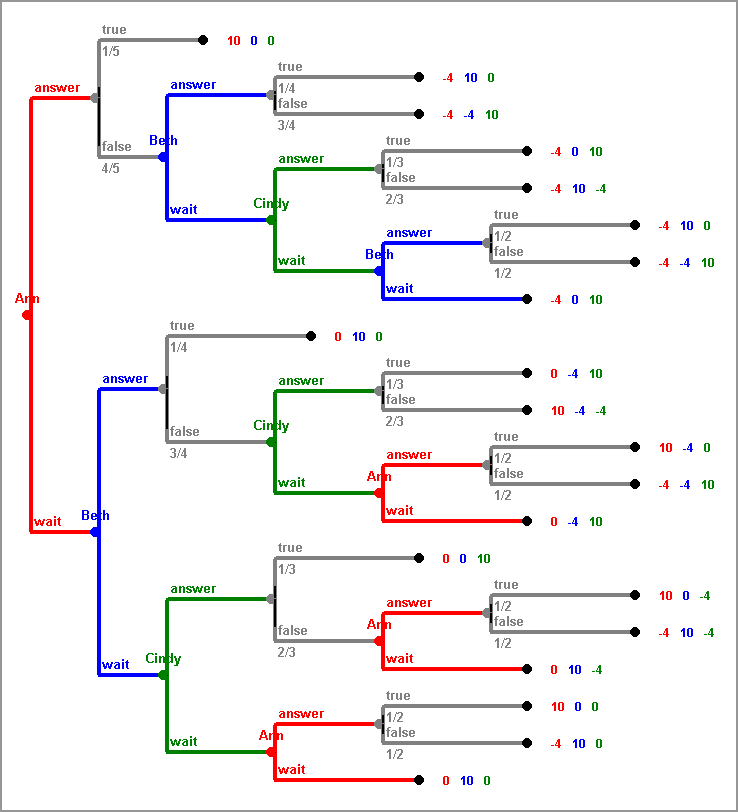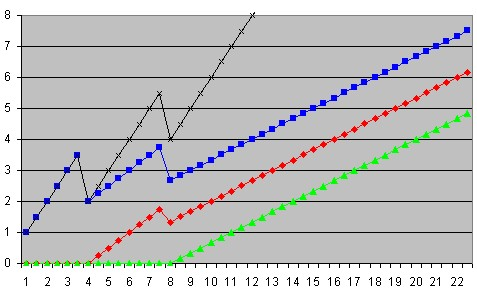MAT109 · Erich Prisner · Franklin College · 2007-2011# Analysis of SEQUENTIAL QUIZ SHOW I

Prerequisites: Chapters 1, 3, 4, and 5.

Pre-class Activity: Every student should bring a very very hard multiple-choice question with 5 options for answers from other classes.

SEQUENTIAL QUIZ SHOW(n,m): Three players, Ann, Beth, and Cindy, are facing a very difficult multiple choice question with five options. Starting with Ann and continuing cyclically, the player on focus can either try to give an answer or wait. If the player tries an answer and the answer is correct, the player gets \$n. If it is incorrect, the player has to pay \$m and is out of the game (cannot try an answer later). If the player waits, the quiz master reveals a wrong answer (thereby decreasing the number of possible options by one) and the next player gets the focus.

Class Activity: We play the game several times, using the questions and answers the students brought to class.

## 1. Candidates with little knowledge

It is rather easy to play this game well: Just give the right answer when it is your turn to answer. In the remainder of this chapter we try to answer the question of how to play this game if the players don't know the right answer. To be more precise, we make the assumption that the question is incredible difficult, so difficult that all three candidates don't have the slightest idea which of the five options could be the right answer. They don't even have suspicions, or can rule out some answers more or less. So, to all three candidates, each of the five possible answers could be right with probability 1/5. All they can do is guess.

Although the procedure of revealing some wrong answers by the show master may remind some of you of the famous (notorious) Monty Hall paradox, it is not really related to it. After a possible answer has been revealed as wrong, either by a candidate betting on it or by the show master when a candidate waits, the probability of being right increases for all remaining possible answers uniformly. This is different in the Monty Hall paradox. Thus, whenever a candidate faces, for instance, three possible answers, each one could be right with probability 1/3. When trying one of them, the candidate will fail with probability 2/3.

The three players move one after the other, so this game is very obviously sequential. The simplest way to describe it, using the assumption of the three candidates not knowing the answer but also knowing that the others don't know, is as follows: First Nature decides randomly, with probability 1/5 for each option, which one of the five possible answers is the right one. Then Ann moves and has six options: Trying answers A, B, C, D, or E, or waiting. In case she tries the answer, the game is either over, if she was right, or Ann lost and is out of the game. In the other case, if Ann waits, one false answer is removed. This is again done by Nature with equal probability for all false answers. In any case, next Beth would have five options. She could try one of the remaining four answers, or wait, and so on. Unfortunately this description of the game is one without perfect information. The human players, Ann, Beth, Cindy, don't know what move Nature did in the first move. We will see later how both formulation in extensive form and analysis can still be done, but for the moment we rather try to describe the game differently, this time with perfect information.

The extensive form of the game is shown below:For SEQUENTIAL QUIZ SHOW(10,4), using expected values and backward induction, it is relatively easy to deduce that Ann and Beth should both wait, and beginning with Cindy everybody just tries any answer. The expected payoff for Ann is 2, for Beth it is 10/3, and for Cindy it is 2/3. See .

In SEQUENTIAL QUIZ SHOW(6,4) all three players Ann, Beth, Chris, should wait. Ann would try to answer it in the second round (with only two options open). Expected payoffs are 1, 3, and 0 for Ann, Beth, and Cindy.

In this Excel Sheet, the payoffs for the different versions of SEQUENTIAL QUIZ SHOW(n,m) are changed automatically, and the game is solved.

### 1.1 More may be less

Assume the candidates have been selected for next day's show. They even know that Ann will start, and Beth will move second. The rules are \$750 for a right answer and a loss of \$400 for a wrong one (i.e. we are playing SEQUENTIAL QUIZ SHOW(750,400)). At the joint dinner with candidates and the sponsor of the show, this sponsor surprises her guests with the statement that she intends to raise the money paid for the right answer to \$810. The three candidates should be pleased to hear this, right?

Not really. After the few moments the (rather bright) candidates need to recalculate the situation, two of the three candidates, Ann and Beth, beg the donor not to increase the prize. Cindy says dully that she doesn't care much, she would rather have another beer. She has been grumpy the whole evening anyway. How could they decline the donor's generous offer?

The answer is simple, if you calculate the old and new version, using the Excel Sheet again. Ann's expectations would be reduced from \$175 to \$137, Beth's expectations would be reduced from \$375 to \$270, only Cindy's expectations would increase very slightly from \$0 to \$3, too small an increase to make Cindy very enthusiastic about the change. But still, this change, and the prospect of a positive expectation it carries, would cause Cindy to change her strategy. Instead of passing when it is her turn, Cindy would try an answer with the increased prize of \$810. This then causes the huge loss for Ann and Beth.

Note however that it is crucial that we talk about non-cooperative games here. If the players can cooperate, are able to discuss the game before it starts, and make binding and enforceable contracts about how to play and side payments, then of course Ann and Beth could promise Cindy a small amount of money if she would keep her passing strategy. Then all three would profit from the increased prize. But if the game were cooperative, all three would play differently from the very beginning. Everybody would pass until Beth, at her second chance, would face only one remaining answer. And then the three would split the prize according to a formula they would have to find. Finding such a formula which is perceived "fair" by all players would be the content of cooperative game theory, of which a glimpse is given in the second part..The graph to the right displays Ann's (in red), Beth's (in blue), and Cindy's (in green) expected payoffs when playing SEQUENTIAL QUIZ SHOW(n,4) with n varying between 1 and 22. The sum of the three expected payoffs is displayed in black. There are two cases where strategies change and therefore the sum of the expectations drop, even for increasing prize value. One is for n=4, and the other for n=8, as discussed above. But note that Beth's position of moving second is always preferable to the other two.

## 2. One candidate knows more

Next assume that at the beginning of the show, just when the question and the possible answers have been posed, one of the players exclaims: "I know one of the answers to be wrong". What changes?

Let us assume first that this player is not lying, and also that the other players believe her. If she is lying, or if there is doubt, or if she knows one of the answers to be false but doesn't tell the others, things get more complicated. Then we get games of incomplete information. These are essentially games where not all players have full knowledge about the structure of the game or the other player's payoffs in the different outcomes. We will discuss some special cases later, but instead of providing general methods of how to tackle games like this, we will only discuss our special example, using ad-hoc methods.

At the beginning, when still all five possible answers are there, the clever candidate has some advantage over the others. A little later, when one of the possible answers has already been deleted, the clever candidate still may maintain the advantage, provided the deleted answer is not the one the candidate privately knows to be false. But it is also possible that exactly this answer has been deleted---then the advantage of the clever candidate is gone. Both situations are possible, and which one occurs is totally decided by luck, the Nature player. Also for the situations with three or two answers left the clever candidate may still have her advantage, or may have lost it, and again which situation occurs depends on luck alone. We want to find out how likely each of the situations is.

Before we look closer into the probabilities for these situations, note that the other two players cannot learn anything of worth from the behavior of the clever player having the additional information. From her action, whether she passes or attempts an answer, they may learn whether the answer the clever candidate knows to be wrong is still on the list, but that doesn't help them to find out the right answer.

Surprisingly it turns out that these probabilities for the clever candidate maintaining her advantage when only 4, 3, or 2 answers are left, do not depend on whether this clever candidate is Ann, Beth, or Cindy. Whenever an answer is eliminated, it is either since the clever candidate tried an answer. But then these probabilities are not relevant anymore---the player with the advantage has either won or is out of the game. Or otherwise the answer is eliminated by another player trying this answer, or by Nature since some player passed. In any case, Nature or the other players would eliminate this answer in question with probability 1/n, if n answers are left.The situation can be described by the probability digraph shown to the right. There are 7 relevant situations, denoted as "nK" or "nN" with n=5,4,3,2. The n denotes the number of answers left, "K" denotes that the clever player still has her advantage, and "N" that she has lost it, since the answer she knows to be wrong is no longer in the list. State "5N" is impossible. Starting with state "5K", the states "4K" or "4N" could emerge. As note above, the transition to "4K" has probability 3/4 and to "4N" has probability of 1/4. In the same way, from situation "4K", situation "3K" will occur with probability 1/3 and situation "3N" with probability 1/3, and from situation "3K", situation "2K" will occur with probability 1/2 and situation "2N" with probability 1/2. On the other hand, if the advantage is already lost, from situations "4N" and "3N" only situations "3N" respectively "2N" can result. The numbers inside the circles representing these situations are the probabilities for them. Starting with a probability of 1 for state "5K", we go forward and compute all other probabilities, using the method discussed on the page on Probability.

In conclusion, the player knowing initially one answer to be wrong maintains her advantage in 3/4 of the cases when there are only 4 answers left, in half of the cases when there are 3 answers left, and in 1/4 of the cases if there is only one answer left.

Now the game tree has to be modified by including a move of Nature before each move the clever player does, provided the player didn't lose the advantage before. These modified DAGs can be found in the three cases where Ann, Beth, or Cindy have this additional information on three different sheets of the same Excel Sheet mentioned already above. Let us discuss the results for the three cases:

### 2.1 Cindy knows one answer to be falseTo the right, the expected payoffs are again displayed, with the prize value as independent variable. Still Beth can expect most, followed by Ann and Cindy, but Cindy's expectations are now closer to Ann's, even passing it for prize value larger than around 19. Interestingly the sum of all three expected values does not differ much from the previous situation. For prize value > 4 it is now 0.25 higher, and otherwise 0.25 lower. Therefore, the gain in expectation Cindy faces, must be balanced somehow by losses of Ann and Beth, or both. It turns out that both lose, but Beth even a little more, and that both gain for Cindy and loss for Ann and Beth increase respectively decrease for increasing prize value. The full picture is given below.

Next we will discuss how wise it was for Cindy to reveal that she knows one answer to be false. If she knows but doesn't reveal this at the beginning, we get a game of incomplete information. ... In fact, it doesn't matter. Both other players would play the same way, in both games. ... WIRKLICH?????????? Therefore convincingly lying that she knows one of the answers to false would also not change the play of the other players, and therefore not affect Cindy's expected payoff. ......... WIRKLICH??????

### 2.2 Ann knows one answer to be false

The modified extensive form can be found on the same Excel file on sheet "cleverAnn". Analysis of this case, using this extensive form, is left to the reader.

### 2.3 Beth knows one answer to be false

This case is left as an exercise.

### Exercises

1. Assume the show master's assistant knows one of the answers to be wrong in advance. In SEQUENTIAL QUIZ SHOW(10,4), to whom would the assistant sell the wrong answer at what price? If you didn't do the previous exercise, just consider the case where Beth would never accept such an illegal hint.
2. It is interesting to note that under the same assumption as in the previous exercise, the assistant could also make money for promising not to reveal the information to anybody. How much money would he get from whom? This is an interesting option: Getting money for not cheating. Again, consider Beth not to be cheating under any circumstances if you didn't analyze the "clever Beth" case before.

### Projects

1. One answer known to be wrong in SEQUENTIAL QUIZ SHOW (n,4):
For this project, you are supposed to use this Excel sheet to generate data. The Excel sheet contains four sheets with the Game Tree in the ordinary case, and in the three cases where one of the players knows one of the answers to be wrong.
1. Assume the show master's assistant knows one of the answers to be wrong in advance. To whom would the assistant sell the wrong answer at what price? Does it depend on n, the value of the prize for winning the quiz show?
2. It is interesting to note that under the same assumption as in the previous exercise, the assistant could also make money for promising not to reveal the information to anybody. How much money would he get from whom? This is an interesting option: Getting money for not cheating.
You are supposed to create data, tables and charts, using the Excel sheet, and also to throroughly describe this data and the conclusions you draw from it with respect to the questions above. You may also try to make sense of it? Are the answers obvious? Are they supported by common sense? What observations and considerations could explain these answers.
2. Coalitions in SEQUENTIAL QUIZ SHOW (n,4):
In Lugano and Como the game is played a little different. In both cases, it is allowed that, before the show starts, two of the three players declare that they have formed a coalition. Then the game is played as usual, but the two players with the coalition must share their win or loss. For instance, if one tried and answer which was wrong, and the other one tried an answer which was right, then both these players receive (n-4)/2.
• Would the members of the coalition expect more than they would if each would play alone? Why? How much more? Does it depend on who forms the coalition? Does it depend on n?
• Would the third player, the one not in the coalition, expect less than if all three would play for themselves? How much less?
• Which coalition is most likely to form?
• The Como variant is still played a little differently. At the beginning of the play, if there is a coalition, they have to declare a ratio, like 50:50 or 60:40, how they would divide wins and loss among themselves. So one player would take 60% of the win, but if they lose money, 60% of it would also be paid by that player. What do you think, which coalition would form in the Como variant, and what ratio would they declare?
• Discuss these questions for different n, like n=10, n=8, n=6, n=12.